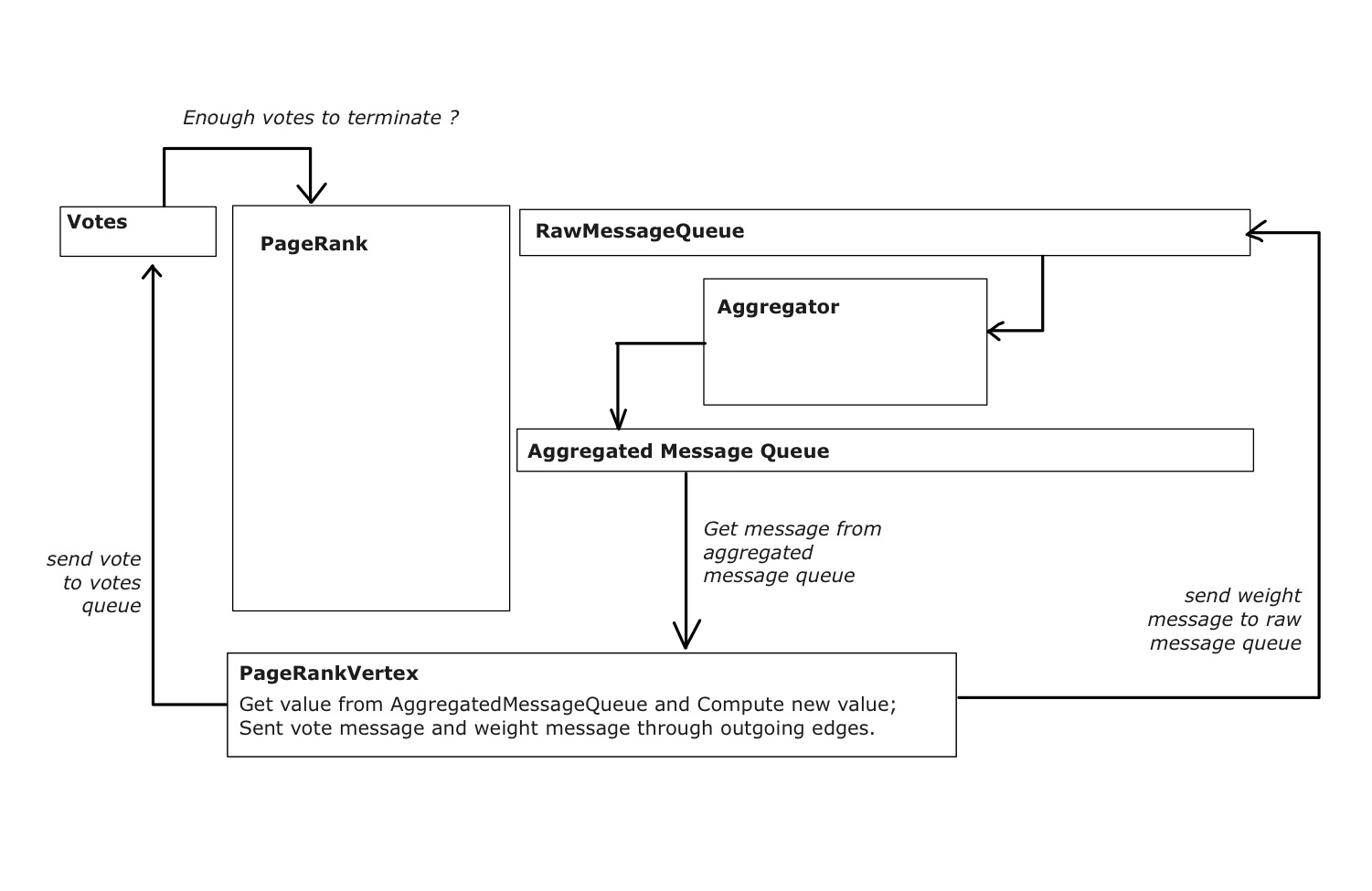# Introduction

This is a project I am doing for a course. The project’s goal is to simulate computing PageRank in a Pregel like system with multithreading in Java.

# Some Basic Information

Pregel Paper

## Message Queue

Message Passing with Threads & Queues

### Vertex

• compute() woule be executed at each active vertex in every superstep.
• compute() is allowed to query information about the current vertex and its edges and to send messages to other vertices.
• compute() can inspect and modify the values of out-edges using methods supplied by the out-edge iterator.
• The values associated with the vertex and its edges are the only pre-vertex state that persists across supersteps.

### Message Passing

• Vertices communicate directly with one another by sending messages.
• A message consists of a message value and the name of the destination vertex.
• Messages sent to vertex $V$ in superstep $S$ are available via an iterator, at superstep $S+1$.
• There is no guarantee on order of messages in the iterator, but it is guaranteed that messages will be delivered and that they will not be duplicated.

### Aggregators

• Each vertex can provide a value to an aggregator in superstep $S$, the system combines those values using a reduction operator.
• Resulting value is made available to all vertices in superstep $S+1$.
• Define a new aggregator by creating a subclass of Aggregator, which is a predefined class. And specify how the aggregated value is initialized from the first input value and how multiple partially aggregated values are reduced to one.

In PageRank task, I am considering using Aggregator’s subclass instance(maybe a function is enough for this project) to aggregate message values sent to the same vertex.

### Worker Implementation

• The state of each vertex contains:
• current value
• a list of outgoing edges
• a queue containing incoming messages
• a flag specifying whether the vertex is active

When the worker performs a superstep it loops through all vertices and calls compute(), passing it the current value, an iterator to the incoming messages, and an iterator to the outgoing edges.

• Two copies of the active vertex flags and the incoming message queue exist: one for the current superstep and one for the next superstep

### Master Implementation

Primarily, the master is responsible for coordinating the activities of workers. Most master operations, including input, output, computation, and saving and resuming from checkpoints, are terminated at barriers.

### Aggregator Implementation

An aggregator computes a single global value by applying an aggregation function to a set of values that the user supplies. When a worker executes a superstep for any partition of the graph, the worker combines all of the values supplied to an aggregator instance into a single value.

# My Thoughts

## Structures## PageRank Class

• Initialize edges; create vertices; set superstep to zero
• Start all vertices’ threads
• While number of vertices that voting to halt is smaller than size
• clear votes; clear rawMessageQueue; clear AggregatedMessageQueue
• superstep += 1
• Send message to all vertices for starting next superstep
• while voteCount is smaller than size
• Count votes received and add it to voteCount
• Aggregate rawMessageQueue into AggregatedMessageQueue
• Count number of -1 in votes

## Vertices

• Up on start, halt
• waiting for a message from Master that indicates starting of next superstep
• get the aggregated message from the in-message-queue.
• Tallcompute(), where the new weight, messages' value,error, vote message would be computed or generated.
• Pause thread and wait for next superstep to start

## Message Class

Class Message is used for holding message information. It has several attributes:

• type: Int
• from: Integer
• to: Integer
• value: Double
• isVote: boolean
• vote: Int

where, type indicates that if this message is a vote or a message that passing weight; from, to marks the sender and receiver of this message; value holds the weight, would be null if message is a vote; isVote is a flag for marking votes message; vote indicates whether the vertex is voting to halt or not.

## Compute() function

• Calculate new weight:
• Calculate message value:
• Send $M$ to all its outgoing edges’ destination
• Calculate error:
• Vote to halt (1 for voting to halt, -1 otherwise)
• Wait on superstep

Note that initially, every vertex’s weight would be set to $\frac{1}{\#vertices}$. Also, in the very first superstep, the error shouldn’t be computed because there are no $R_{previous}$ value available.

Updated: